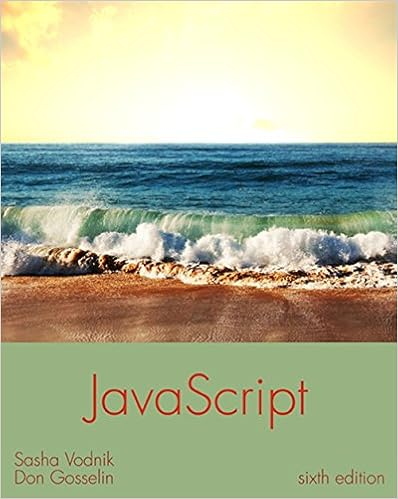# What planet is the biggest string choicessize a

• Homework Help
• 46
• 97% (176) 170 out of 176 people found this document helpful

This preview shows page 43 - 46 out of 46 pages.

##### We have textbook solutions for you!
The document you are viewing contains questions related to this textbook.The document you are viewing contains questions related to this textbook.
Chapter 2 / Exercise 4
JavaScript: The Web Warrior Series
VodnikExpert Verified
##### We have textbook solutions for you!
The document you are viewing contains questions related to this textbook.The document you are viewing contains questions related to this textbook.
Chapter 2 / Exercise 4
JavaScript: The Web Warrior Series
VodnikExpert Verified
Programming Logic and Design, 8e Solutions 9- num displayAnswer(string guess, string answer) Declarations num correct = 0 if guess = answer then output “Correct!” correct = 1 else output “The correct answer is: ”, answer endif return correct 2. In the Game Zone section of Chapter 6, you designed the logic for the game Hangman, in which the user guesses letters in a hidden word. Improve the game to store an array of 10 words. One at a time, pass each word to a method that allows the user to guess letters continuously until the game is solved. The method returns the number of guesses it took to complete the word. Store the number in an array before returning to the method for the next word. After all 10 words have been guessed, display a summary of the number of guesses required for each word as well as the average number of guesses per word. 44
Programming Logic and Design, 8e Solutions 9- Declarations character letter num numCorrect num length num x num guesses // get the length of the word length = 0 while word[length] exists length = length + 1 endwhile // display dashes “-” x = 0 while x < LENGTH output “-” endwhile numCorrect = 0 output “Please guess a letter” input letter guesses = 1 while numCorrect <> length x = 0 while x < length if word[x] = letter then numCorrect = numCorrect + 1 output word[x] else output “-” endif x = x + 1 endwhile output “Please guess a letter” input letter guesses = guesses + 1 endwhile output “You guessed the word!” output word return guesses Up for Discussion
•••